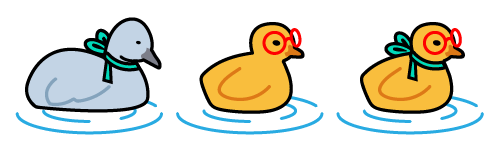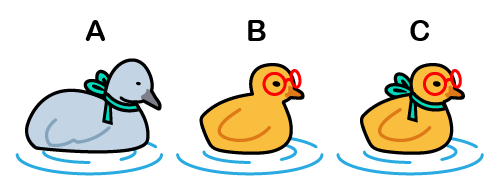# Ugly Duckling Theorem Calculator

Created by Luciano Miño
Reviewed by Anna Szczepanek, PhD and Adena Benn
Based on research by
Satosi Watanabe Knowing and guessing, a quantitative study of inference and information New York, Wiley (1969)
Last updated: Jun 14, 2023

This ugly duckling theorem calculator can aid you in understanding the implications of showing the differences and similarities between any two objects.

Although it may appear simple initially, this theorem shows that there's really no ugly duckling if we remove all bias from the classification process.

In this text, we will show:

• What Watanabe's ugly duckling theorem means;
• The relation between the ugly duckling theorem and pattern recognition;
• What the Hamming distance is; and
• An example of visualizing this problem.

## What is the ugly duckling theorem?

The ugly duckling theorem asserts that classification without bias makes all objects equally similar and dissimilar. It originated in Satosi Watanabe's "Ugly duckling theorem" from "Knowing and Guessing, A Quantitative Study of Inference and Information (1969)".

In the original story "The Ugly Duckling" by Hans Christian Andersen, a duckling struggles to find its place due to the apparent differences between it and its duck family. The story concludes when the duckling realizes it wasn't a duck but a swan the whole time.

According to this theorem, two ducklings share the same number of similarities between them as any of them share with the baby swan.

## Explaining the theorem

Assume we have three objects A, B, and C, and we want to find the one which differentiates the most from the others.

For that, we will separate them using the list of all boolean functions that arise from $m$ initial features.

Let's say that initially, we separate the objects based on whether they have legs or wings and call these classes $L$ and $W$, respectively. Note that you can use any feature you'd like.

The size of the set of all boolean functions created from these two features is $2^{2m}$, in our case $2^{4} = 8$.

∧ = and, ∨ = or, ¬ = not. For example, L ∧ ¬W means the object has legs AND doesn't have wings.

Boolean functions from $L$ and $W$

L ∧ W

L ∧ ¬W

L ∨ W

L ∨ ¬W

¬L ∧ W

¬L ∧ ¬W

¬L ∨ W

¬L ∨ ¬W

For further reading into logic operators, check our AND calculator, NOR calculator, or XOR calculator.

💡 These boolean functions are created from all possible combinations that arise from applying the logical operators to the initial features. E.g., $¬W ∨ ¬L$ means the object does not have wings or does not have legs.

### Representing each object by a n-bit string

Therefore, we can use a string of $8$ bits to represent each object by assigning each digit to each boolean function.

E.g., $1 0 1 1 0 0 1 0$

To avoid bias in this representation, each digit will have the same importance as the others when comparing the objects.

Lastly, we will take the order of the digits from the list above. An object containing $1$ on the leading digit will have both legs and wings.

### The Hamming distance

The Hamming distance is a way to compare two binary strings of equal length by counting the number of positions where their bits differ. For example, $1100$ and $1101$ would have a Hamming distance of $1$.

We can use the Hamming distance in our problem to find the most dissimilar object. Let's do that!

### Comparing the n-bits string objects

Let's say we've paired each object with its corresponding n-bits string as follows:

$A = 1\ 0\ 1\ 1\ 0\ 0\ 1\ 0$
$B = 0\ 1\ 1\ 1\ 0\ 0\ 0\ 1$
$C = 0\ 0\ 1\ 0\ 1\ 0\ 1\ 1$

Look at each and count how many bits $A$ and $B$ have in common. Now compare $A$ and $C$.

No matter which strings you choose to compare; they will all have four bits in common and four different bits.

Since each bit refers to a "feature", and each of these features is as important as the others, Watanabe's ugly duckling theorem concludes that we can't really say that these objects are more similar than they are dissimilar.

The only way to distinguish them would be to consider any of the features more appealing than the others. This, however, introduces bias.

🙋 Use the ugly duckling theorem calculator to understand this example with a more visual representation 🦆.

## FAQ

### How do Watanabe's ugly duckling theorem and pattern recognition relate?

Watanabe's ugly duckling theorem states that without bias, all objects are equally similar and dissimilar when compared to each other. For pattern recognition, this means that the objects' features should be weighted accordingly, taking into account the particular problem to be solved.

### What is the Hamming distance of 01 and 10?

2. The Hamming distance of 01 and 10 is the number of positions in which their bits differ. Since we need to switch two bits from 01 to get to 10, the Hamming distance is 2.

### How do I calculate the Hamming distance?

To calculate the Hamming distance of any two equal-length bit strings:

1. Compare the first bit of each of the strings.
2. Repeat comparing each position until you've covered the whole string, and count the number of times these bits differ.
3. The result is the Hamming distance between the strings.
Luciano Miño
The ugly duckling theorem
The ugly duckling theorem states that classification without any sort of bias makes any two objects equally similar and equally different from each other.
Which one is the ugly duckling?
Let's picture this with an exercise. Suppose there are three ducklings, and we want to differentiate them from one another without any sort of bias as to which features are more important than others.We will refer to the set of ducklings with a scarf as S, and the set of ducklings with glasses as G.

Let's classify each duckling separately.
Comparison table
We will write a table using all possible boolean functions to list all the "features" a duckling may have (remember we are trying to avoid any sort of bias, so having a scarf is as valuable as not having one).

After that, if we count the number of features each duckling has and compare them, we will find the duckling with the most differences.

We went ahead and completed the column for duckling A. Write down the table and finish the other two columns putting '1' if the duckling fulfils the criteria or '0' if it does not.

For example, S ∧ ¬G means the duckling has a scarf AND doesn't have glasses.

Afterwards select "yes" to show the completed table and compare with yours.Show completed table
No
∧ = and, ∨ = or, ¬ = not.
ABC
S ∧ G0--
S ∧ ¬G1--
S ∨ G1--
S ∨ ¬G1--
¬S ∧ G0--
¬S ∧ ¬G0--
¬S ∨ G0--
¬S ∨ ¬G1--
People also viewed…

### Alien civilization

The alien civilization calculator explores the existence of extraterrestrial civilizations by comparing two models: the Drake equation and the Astrobiological Copernican Limits👽

### Decagon area

Use this decagon area calculator to find the area of a decagon using any easy-to-measure parameter of a decagon.

### Digital root

This digital root calculator finds the single-digit sum of all digits in a number after iterative additions.

### Lost socks

Socks Loss Index estimates the chance of losing a sock in the laundry.